﻿ 基于医学图像纹理特征量优化的肝纤维化分级 Staging of Hepatic Fibrosis Based on Optimization of Selected Texture Features

Computer Science and Application
Vol.08 No.07(2018), Article ID:26029,13 pages
10.12677/CSA.2018.87121

Staging of Hepatic Fibrosis Based on Optimization of Selected Texture Features

Ganxin Ouyang1, Xuejun Zhang1,2*, Dongbo Wu3

1School of Computer, Electronics and Information, Guangxi University, Nanning Guangxi

2Guangxi Key Laboratory of Multimedia Communications and Network Technology, Guangxi University, Nanning Guangxi

3Liuzhou Worker’s Hospital of Guangxi Zhuang Autonomous Region, Liuzhou Guangxi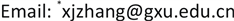Received: Jul. 1st, 2018; accepted: Jul. 16th, 2018; published: Jul. 23rd, 2018ABSTRACT

By now, it is still lack of guidance conclusion on texture features selection and image modalities optimization for the computer-aided diagnosis of hepatic fibrosis. Based on the extraction of texture features from large amount of MRI and CT images, lack of research exists on computer-aided diagnosis of hepatic fibrosis on medical images, this paper is to study the optimization of computer-aided diagnosis program of hepatic fibrosis, we can not only conclude options on Regions of Interest of hepatic MRI and CT images, but also get optimal selection of medical image features using SVM algorithm classifier; we find that the optimal experiment performance is equilibrium phase and 20 × 20 pixels size of ROI; the optimal number of features is confirmed from 3 to 7. The weighted values of 15 features for computer aided diagnosis of hepatic fibrosis in hepatic MRI and CT images are calculated and ranked; the hepatic MRI images reflect the degree of liver fibrosis is better than CT images; the distribution of effective features in liver MRI images is more concentrated than that in liver CT images.

Keywords:Computer-Aided Diagnosis, Medical Images, Hepatic Fibrosis, SVM Algorithm Classifier

1广西大学计算机与电子信息学院，广西 南宁

2广西大学多媒体通信与网络技术重点实验室，广西 南宁

3广西壮族自治区柳州市工人医院，广西 柳州1. 引言

2. 分类诊断实验

2.1. 实验材料的提取

2.1.1. 医学图像材料

2.1.2. 感兴趣区域的选取

2.1.3. 纹理特征提取

2.2. 分类诊断实验方法

2.2.1. 穷举遍历法

${C}_{n}^{1}+{C}_{n}^{2}+{C}_{n}^{3}+\cdots +{C}_{n}^{n}={2}^{n}-1$ (2-1)

2.2.2. 留一法

2.3. 实验流程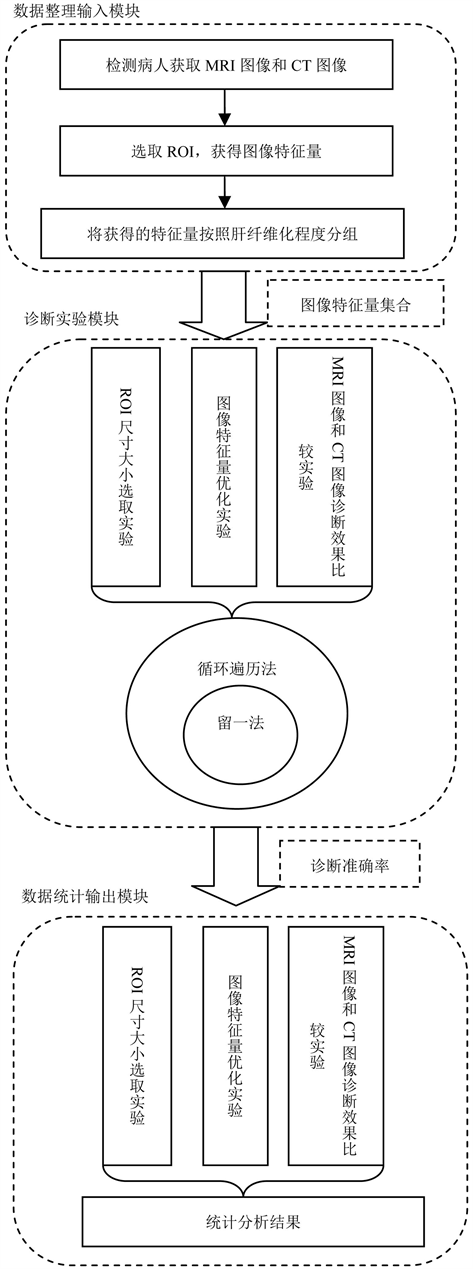Figure 1. Flow chart of diagnostic classification

3. 实验数据统计及分析

3.1. ROI尺寸选取

${P}_{\text{MA}}=\frac{1}{6}\sum _{n=1}^{6}{P}_{\mathrm{max}}\left(n\right)$ (3-1)

Table 1. (a) The highest average accurate rate distribution of classification experiment with MRI liver images; (b) The highest average accurate rate distribution of classification experiment with CT liver images

3.2. 图像特征量的优化

3.2.1. 图像特征量个数优化

${P}_{\text{ma}}\left(k\right)=\frac{1}{72}\sum {P}_{\mathrm{max}}\left(k\right)\text{\hspace{0.17em}}\text{\hspace{0.17em}}\left(k=1,2,3,\cdots ,15\right)$ (3-2)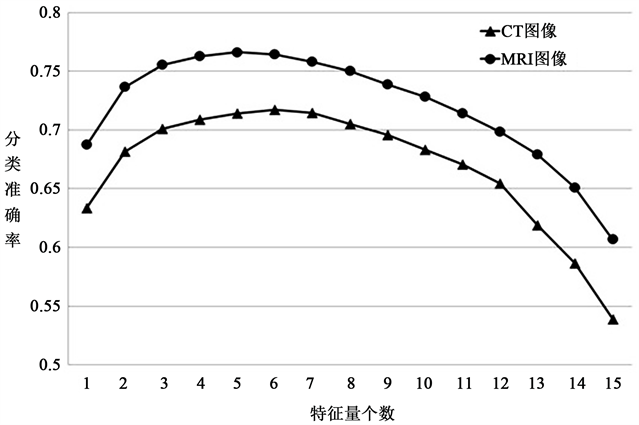Figure 2. The curve of the number of MRI and CT images features optimal selectingTable 2. The optimal selection of the number of MRI and CT images features

3.2.2. 图像特征量组合优化

$f\left(x\right)=\frac{1}{\sqrt{2\text{π}}\sigma }{\text{e}}^{-\frac{{\left(x-\mu \right)}^{2}}{2{\sigma }^{2}}},\text{\hspace{0.17em}}0 (3-3)

$\left\{\begin{array}{l}{P}_{\text{MRI}}\left(\text{features}\right)\ge {\mu }_{\text{MRI}}\\ {P}_{\text{CT}}\left(\text{features}\right)\ge {\mu }_{\text{CT}}\end{array}$ (3-4)

${C}_{\text{ASM}}=\text{Normalized}\left(\sum {P}_{\text{MRI}}\left(\text{features}\right)\right)$

$\text{ASM}\in \text{features}$ (3-5)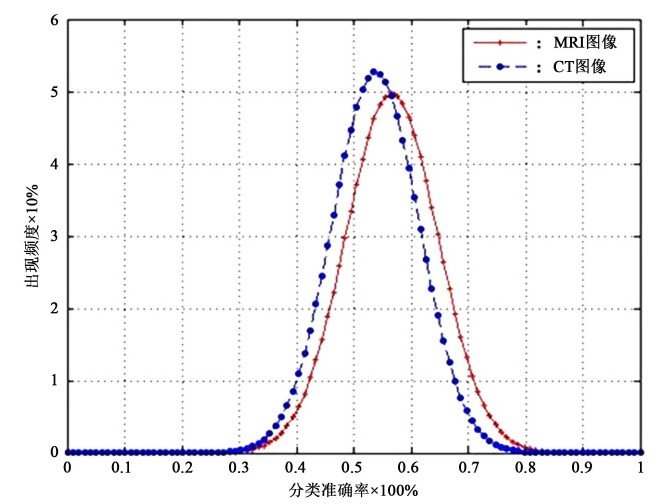Figure 3. The distribution of classification accurate rate of MRI and CT images features

${C}_{\text{ASM}}=\text{Normalized}\left(\sum {P}_{\text{CT}}\left(\text{features}\right)\right)$

$\text{ASM}\in \text{features}$ (3-6)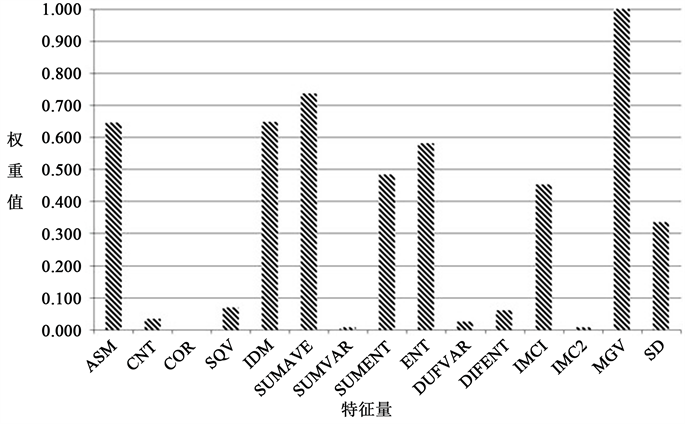Figure 4. The distribution curve of MRI images features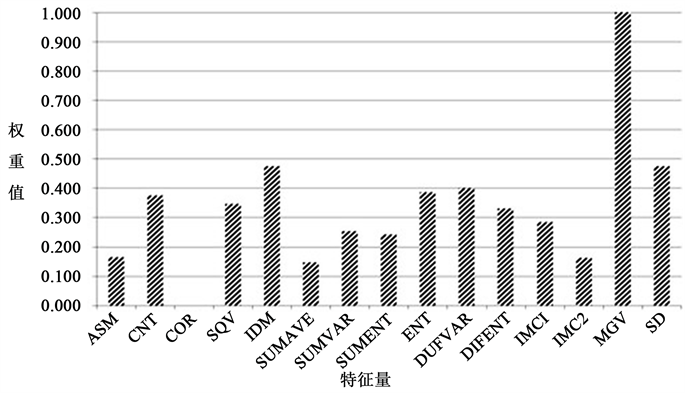Figure 5. The distribution curve of CT images features

Table 3. (a) Weight value of MRI and CT images features (1); (b) Weight value of MRI and CT images features (2)

Table 4. (a) The ranking of MRI liver images features weight value; (b) The ranking of CT liver images features weight value

3.3. MRI和CT图像的诊断实验比较

${\mu }_{C}=\frac{1}{15}\sum _{i=1}^{15}{C}_{i}$ (3-7)

${\sigma }_{C}=\sqrt{\frac{1}{15}\sum _{i=1}^{15}\left({C}_{i}-{\mu }_{C}\right)}$ (3-8)

4. 结论

Staging of Hepatic Fibrosis Based on Optimization of Selected Texture Features[J]. 计算机科学与应用, 2018, 08(07): 1089-1101. https://doi.org/10.12677/CSA.2018.87121

1. 1. 王惠明, 史萍. 图像纹理特征的提取方法[J]. 中国传媒大学学报自然科学版, 2006(13): 49-52.

2. 2. 朱福珍, 吴斌. 基于灰度共生矩阵的脂肪肝B 超图像特征提取[J]. 中国医学影像技术, 2006, 22(2): 287-289.

3. 3. Chabat, F., Yang, G.Z. and Hansell, D.M. (2003) Obstructive Lung Diseases: Texture Classification at CT. Radiology, 228, 871-877. https://doi.org/10.1148/radiol.2283020505

4. 4. 曹桂涛, 施鹏飞, 等. 基于肝脏超声图像的纤维化量化分析[J]. 声学技术, 2004, 11(3): 98-104.

5. 5. 陶振中, 景英娟, 董育宁. M PEG-7描述子在医学CT图像CBIR中的应用[J]. 信息技术, 2006(5): 8-19.

6. 6. Sheshadri, H.S. and Kandaswamy, A. (2007) Experimental Investigation on Breast Tissue Classification Based on Statistical Feature Extraction of Mammograms. Computerized Medical Imaging and Graphics, 31, 46-48. https://doi.org/10.1016/j.compmedimag.2006.09.015

7. 7. Jirak, D., Dezortova, M., Taimr, P., et al. (2002) Texture Analysis of Human Liver. Journal of Magnetic Resonance Imaging, 15, 68-74. https://doi.org/10.1002/jmri.10042

8. 8. Jafari-Khouzani, K., Siadat, M.-R., et al. (2003) Texture Analysis of Hippocampus for Epilepsy. Proceedings of SPIE, 503, 279-288.

9. 9. Vittitoe, N.F. and Baker, J.A. (1997) Fraetal Texture Analysis in Computer-Aided Diagnosis of Solitary Pulmonary Nodules. Academic Radiology, 4, 96-101.

10. 10. Ahmed, A.T., Dubois, P. and Duquenoy, E. (2003) Analysis Methods of CT Scan Images for the Characterization of the Bone Texture: First Results. Pattern Recognition Letters, 24, 1971-1982. https://doi.org/10.1016/S0167-8655(03)00036-9

11. 11. 蒋勇. 基于分形维数的肺部软组织CT图像的纹理特征研究[J]. 中国医学装备, 2004, 1(3): 28-31.

12. 12. 中华医学会传染病与寄生虫病学分会、肝病学分会. 病毒性肝炎防治方案[R]. 西安, 2000.

13. 13. Edelman, R.R., Siegel, J.B., Singer, A., Dupuis, K. and Longmaid, H.E. (1989) Dynamic MR Imaging of the Liver with Gd-DTPA: Initial Clinical Results. American Journal of Roentgenology, 153, 1213-1219. https://doi.org/10.2214/ajr.153.6.1213

14. 14. Roche, K.J., Genieser, N.B. and Ambrosino, M.M. (1996) Pediatric Hepatic CT: An Injection Protocol. Pediatric Radiology, 26, 502-507. https://doi.org/10.1007/BF01372229

15. 15. 内山良一, 張学軍, 藤田広志. 形態情報における画像診断－脳と肝臓のMRIによる診断支援技術[J]. 映像情報メディア学会誌, 2011, 65(4): 436-439.

16. 16. 藤田広志, 原武史, 周向栄, 林達郎, 神谷直希, 張学軍, 陳華岳, 星博昭. 計算解剖モデルの構築[J]. Medical Imaging Tech-nology, 2011, 29(3): 10-17.

17. 17. Wu, C. and Chen, Y. (1992) Texture Features for Classification of Ultrasonic Liver Images. IEEE Transactions on Medical Imaging, 11, 141-152. https://doi.org/10.1109/42.141636

18. 18. Zheng, J., Kazunobu, Y., Kentaro, Y., et al. (2006) Support Vector Machine-Based Feature Selection for Classification of Liver Fibrosis Grade in Chronic Hepatitis C. Journal of Medical System, 30, 389-394. https://doi.org/10.1007/s10916-006-9023-2

19. 19. Haralick, R.M., Shanmugam, K. and Dinstein, I. (1973) Textural Features for Image Classification. IEEE Transaction on Systems, Man and Cybernetics, SMC-3, 610-621. https://doi.org/10.1109/TSMC.1973.4309314

20. 20. Justel, A., Peña, D. and Zamar, R. (1997) A Multivariate Kolmogo-rov-Smirnov Test of Goodness of Fit. Statistics & Probability Letters, 35, 251-259. https://doi.org/10.1016/S0167-7152(97)00020-5

21. 21. Abdi, H., Williams, L.J. and Valentin, D. (2013) Multiple Factor Analysis: Principal Component Analysis for Multitable and Multiblock Data Sets. Wiley Interdisciplinary Reviews: Computational Statistics, 5, 149-179. https://doi.org/10.1002/wics.1246

22. NOTES

*通讯作者。